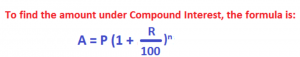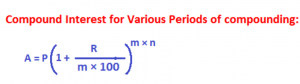Compound Interest is different from a simple interest.

In simple interest, interest is computed on original principal and added to it. In compound interest, interest is regularly added to the principal at the end of the agreed intervals of time to generate a fresh and new principal. Principal, interest for the next interval of time is again computed and added to generate another new higher principal for the next interval of time. Thus, interest is regularly added to principal at regular frequency during the whole time period of investment.Note: Compounding means adding interest to principal

Consider the following example as an illustration for Compound Interest:

Suppose a man invested 1000 at 10% rate of interest compounded annually. What is the amount he gets back at the end of 2 years?

In 1 year, at 10% p.a., 1000 generates an interest amount of 100. This interest amount is added the original principal to create a new and fresh principal for computing the interest for the second year. Therefore, principal for the second year is: 1000 + 100 = 1100.

On this new principal 1100, at 10% p.a., for the next one year of time period, the interest is: (1100 × 1 × 10)/100 = 110.

This interest is again added to its own principal, i.e. 1100. Therefore, amount in 2 years is 1100 + 110 = 1210. Hence, 1000 yields 1210 in 2 years at 10% p.a. compounded annually.In the above example:

P = 1000, R = 10% p.a. n = 2 (time period).

Applying the values in the above formula, we get: A = 100 (1 + 10/100) 2 = 1210

Note:

The above formula is quite famous. But it is applicable only when interest is added to the principal (i.e. compounding) is done annually. Compounding, which is adding interest to the principal, can happen at other intervals of time than annually. Other popular intervals of time for compounding are half-yearly, quarterly and monthly.

What will be the formula to calculate Amount under C.I. for other frequency of compounding?m stands for frequency of compounding, i.e the number times compounding is done within a year.

The following table provides the value of m for different frequency of compounding:Solved Problems on Compound Interest:

Example 1:

The difference between compound interest (compounded annually) and simple interest on a principal P at 10% rate of interest p.a. is 200. Find the sum.

Solution:

Under C.I., amount A compounded annually is: A = P [1 + R/100)] n

We know that compound interest is = A – P i.e. C.I. = P [1 + 10/100)] 2 ― P = (21/100) P and simple interest is: S.I. = (P × 2 × 10)/100 = P/5

Now, write C.I. – S.I. = 200 (as given in the question)

• i.e. (21/100) P – (P/5) = 200
• i.e. (1/100) P = 200
• i.e. P = 20000

Example 2:

Under C.I. compounded annually, a sum of money doubles in 4 years. In how many years will this sum grow four-fold?

Solution:

Under C.I., amount A compounded annually is: A = P [1 + R/100)] n

In the above formula, write A = 2P and n = 4. So, we have:

2P = P [1 + R/100)] 4

i.e. 2 = [1 + R/100)] 4

i.e. [1 + R/100)] = (2)1/4 ………….eq 1

Now, write A = 4P to find n (the required time) in the formula: A = P [1 + R/100)] n

4P = P [1 + R/100)] n

4 = [1 + R/100)] n ……….eq 2

From eq 1, write (2)1/4 in [1 + R/100)] in eq 2

So, we have: 4 = (21/4) n i.e. 22 = 2n/4.

As the base 2 is same on either side, therefore equate the exponents:

i.e. 2 = n/4, i.e n = 8 – so, it takes 8 years